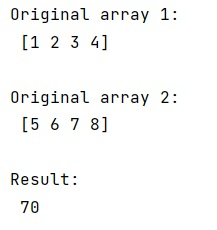# Is there a numpy/scipy dot product, calculating only the diagonal entries of the result?

Learn, how to calculate dot product only on the diagonal entries of the array?
Submitted by Pranit Sharma, on February 16, 2023

NumPy is an abbreviated form of Numerical Python. It is used for different types of scientific operations in python. Numpy is a vast library in python which is used for almost every kind of scientific or mathematical operation. It is itself an array which is a collection of various methods and functions for processing the arrays.

## Calculating only the diagonal entries of the result

Suppose that we are given two numpy array and we are performing some operation on these two. We need to calculate the dot product for the diagonal entries of the result of the operation which we perform on the arrays.

For this purpose, we must use numpy.diag() while calculating the dot product of an array with the other array which is equivalent to the individual sum of the scalar product of rows of the first array and columns of other arrays. Hence we will multiply the rows of the first array with the transpose of other arrays.

Let us understand with the help of an example,

## Python code to calculate dot product only on the diagonal entries of the array

```# Import numpy
import numpy as np

# Creating two numpy arrays
arr = np.array([1,2,3,4])
arr1 = np.array([5,6,7,8])

# Display original arrays
print("Original array 1:\n",arr,"\n")
print("Original array 2:\n",arr1,"\n")

# Calculating dot product along the diagonals
res = (arr * arr1.T).sum(-1)

# Display Result
print("Result:\n",res)
```

Output: